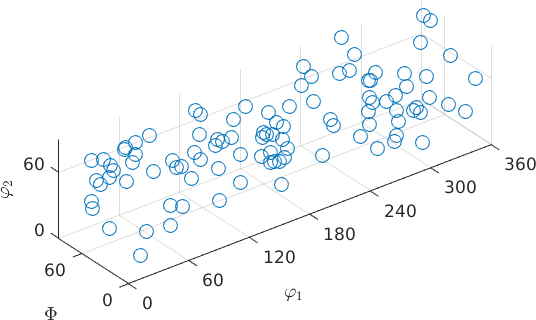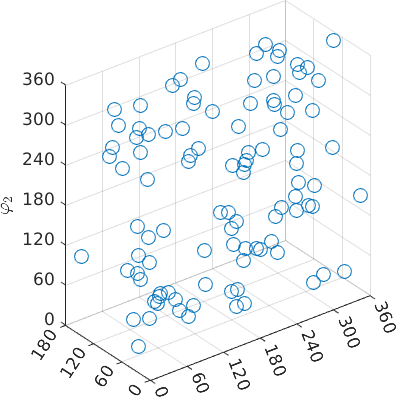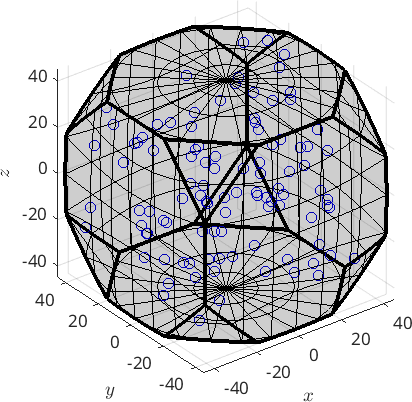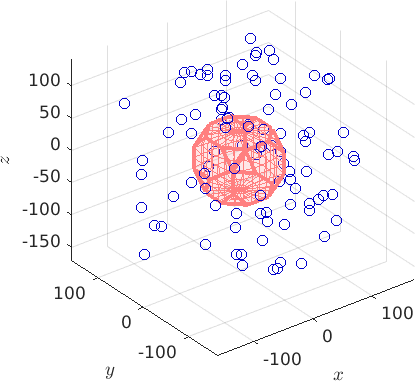3D Orientation Visualizations edit page

## Euler angle space

By default the function plot plots orientations in the three dimensional Bunge Euler angle spaceNote that the orientations are automatically projected into the fundamental region. In the case of cubic symmetry this means that the Euler angles $$\Phi$$ and $$\phi_2$$ are restricted to 90 degrees. If the orientations should be plotted at their specified Euler angles the option 'ignoreFundamentalRegion' has to be used.## Axis angle space

Alternatively, orientations can be plotted in the three dimensional axis angle space.The orientations are automatically projected into its fundamental region. Again, this can be switched off with the option 'ignoreFundamentalRegion'.